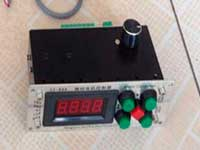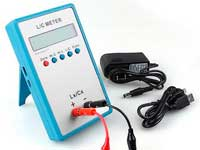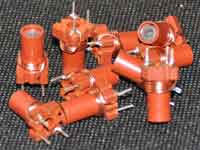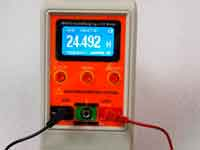Conical Tesla Coil - 5.0 out of 5 based on 2 votes
Rating 5.00 (2 Votes)

Conical Tesla CoilAlthough to put it mildly, I do not share all sorts of metaphysical theories enthusiasts Tesla coils and catchers "free energy ether", that is no reason to abandon the implementation of the calculation of such inductors, especially conical coil, which is often used in such devices. The conical coil can be calculated by the following empirical Harold Wheeler formulas, which Tesla was using:

• N - number of turns
• A - diameter of base of cone
• B - diameter of top of cone
• H - height of cone
• W = (B - A)/2 - effective width of coil
• Z - length of coil (along hypotenuse!) Z = √(W2 + H2)
• X - cone angle (sin(X) = H/Z; cos(X) = W/Z)
• R = A/2 + W/2 - average radius of coil
• L1 = R2*N2/(9*R+10*H) - vertical component of inductance
• L2 = R2*N2/(8*R+11*W) - horizontal component of inductance
• L = √[(L1*Sin(X))2 + (L2*cos(X))2] - aggregate inductance of coil

Inductance in µH, dimensions in inches.

But our calculator uses Maxwell's formula in the same way as the flat spiral coil calculator and the multi-layer coil calculator. The calculator was updated 2018/05/02. Be sure to update the browser cache.

Conical Tesla coil calculatorEnter the input data:
 A = mmcm – The base cone diameter B = mmcm – The top cone diameter H = mmcm – Height of cone dw =  mm – Wire diameter N = – Number of turns

RESULT:
 L = mHµHnH – Inductance

Сomments from anonymous guests are enabled with moderation.

Coil Winding MachineUS \$187.53
The system consists of individual components including a controller, a stepping motor, a foot switch, power adapter and output shaft. This design makes it very easy for you to integrate the system into your own project.

LC Meter LC200AUS \$37.99
Measuring Capacitance Range:
0.01 pF - 100mF
Measuring Inductance Range:
0.001 uH - 100H
Measurement accuracy:1%.

RF InductorsUS \$6.43
Variable Ferrite Core Inductor coil set 3.5Turns 25-100MHZ

Auto Ranging LCR MeterUS \$63.07
Meter can measure 0.00pF to 100.00mF capacitance, 0.000uH to 100.00H inductance and 0.00? to 10.000M? resistance. Very suitable for small value capacitor and inductor measurement.